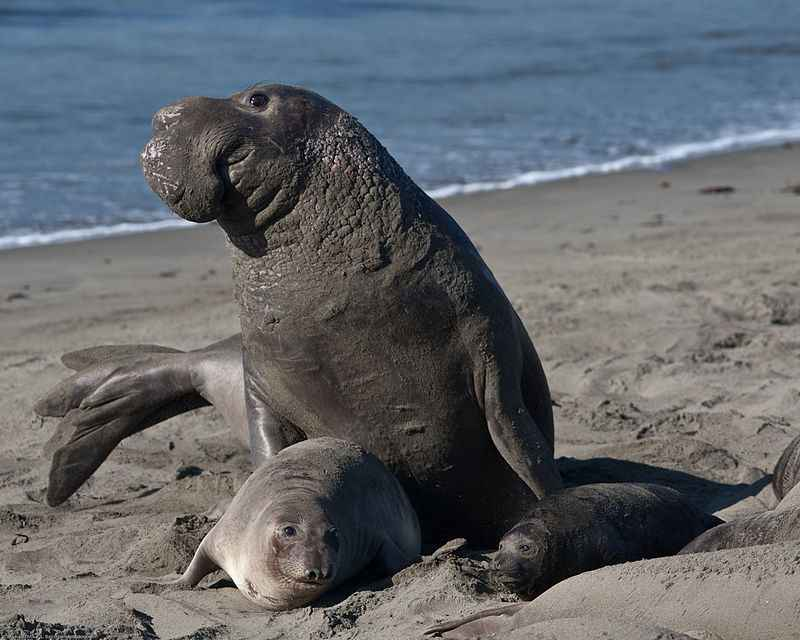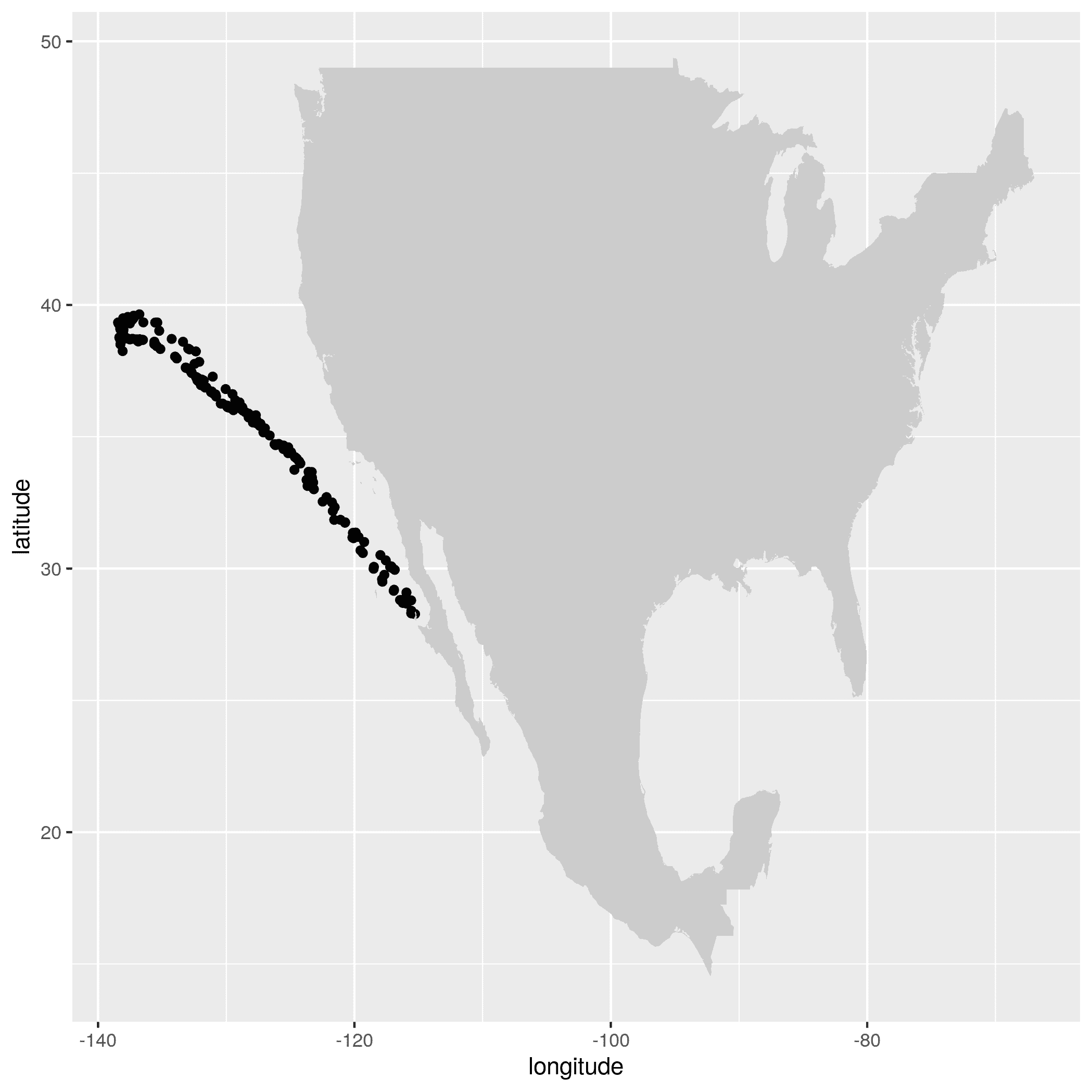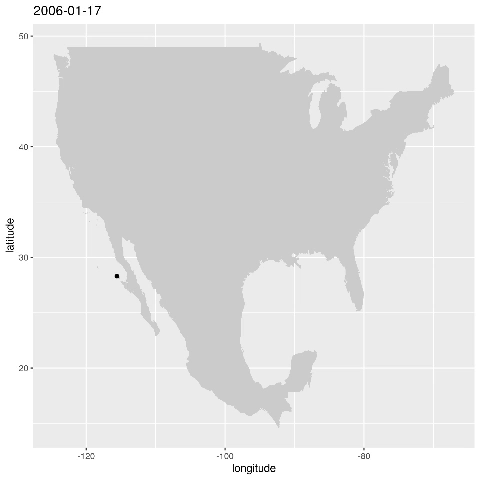There is a lot of easily accessible data online waiting to be explored and analyzed. Recently, I came across a work shop for a U.S. Integrated Ocean Observing System (IOOS) Biological Data Training Workshop. Workshops are great as they usually post their code and slide shows for everyone to see and explore. One of the code examples on the posted github dealt with animal telemetry data - let’s explore the data together. First load the libraries

``````library("rerddap")
library("akima")
library("dplyr")
library("ggplot2")
library("mapdata")
library("ncdf4")
library("plot3D")
``````

ERDDAP is a data server that provides a way to download various scientific data sets. There are lots of various data sets available through IOOS such as trawl surveys, ocean temperatures and others. Coding in `R` throughout this post, we will first specify the animal telemetry data (`gtoppAT`):

``````atnURL <- 'http://oceanview.pfeg.noaa.gov/erddap/'
atnInfo <- info('gtoppAT', url = atnURL)
atnInfo
``````

produces

`````` Variables:
commonName:
isDrifter:
Range: 0, 1
latitude:
Range: -77.891, 77.193
Units: degrees_north
LC:
longitude:
Range: 0.01, 359.971
Units: degrees_east
project:
Range: 0, 1
serialNumber:
time:
Range: 1.02512583108E9, 1.572152616E9
Units: seconds since 1970-01-01T00:00:00Z
toppID:
yearDeployed:
Range: 1995, 9999
``````

which give’s us the data ‘column’ names. Running the command

``````atnData <- tabledap(atnInfo, fields = c("commonName"), url = atnURL)
atnData
``````

gives us the names of all the animals that are avaiable in the data set:

``````<ERDDAP tabledap> gtoppAT
Path: [/home/stan/.cache/R/rerddap/8c470a421cf8d42a59fd20a943fc89dd.csv]
Last updated: [2019-10-28 17:11:13]
File size:    [0 mb]
# A tibble: 53 x 1
commonName
<chr>
1 Atlantic Sailfish
3 Bigeye Tuna
4 Black Marlin
5 Black-footed Albatross
6 Blue Marlin
7 Blue Shark
8 Blue Whale
9 California Sea Lion
10 Common Thresher Shark
# … with 43 more rows
``````

There are `53` species tracked in the database. Let’s track the one with the largest `toppID` count

``````res <- tabledap(atnInfo, fields = c("toppID"), 'commonName="Atlantic Sailfish"', url = atnURL)
maxLength <- length(res\$toppID)
maxName <- "Atlantic Sailfish"
for (name in atnData\$commonName) {
cond<-paste('commonName="',name,'"',sep="")
res <- tabledap(atnInfo, fields = c("toppID"), cond, url = atnURL)

if(maxLength < length(res\$toppID)){
maxLength = length(res\$toppID)
maxName = name
}
}
print(paste(maxName,"has the maximum toppID count of",maxLength))
``````

returns

`````` "Northern Elephant Seal has the maximum toppID count of 387"
``````

Here’s a picture of the North Elephant Seal from Wikipedia:There are a total of `146,668` data entries for the Northern Elephant Seal. Let’s focus on `toppID=2006008`:

``````res <- tabledap(atnInfo, fields = c("time","longitude", "latitude","toppID"), 'commonName="Northern Elephant Seal"','toppID="2006008"', orderby="time",url = atnURL)
res\$longitude <- as.numeric(res\$longitude)
res\$latitude <- as.numeric(res\$latitude)
xmin<-min(res\$longitude-360)
xmax<-max(res\$longitude-360)
ymin<-min(res\$latitude)
ymax<-max(res\$latitude)

w <- map_data("worldHires", ylim = c(ymin,ymax), xlim = c(xmin,xmax))
for
alldata <- data.frame(longitude = res\$longitude-360, latitude = res\$latitude)
ggplot() + geom_point(data=alldata,aes(x=longitude,y=latitude)) + geom_polygon(data = w,aes(x=long,y=lat,group=group),fill="grey80")
``````

This produces the figure that shows where a specific seal visisted during the time period 2006-01-17 to 2006-05-16:Let’s animate the results

``````for (i in 1:length(res\$time)){

alldata <- data.frame(longitude = res\$longitude[1:i]-360, latitude = res\$latitude[1:i])

plot = ggplot() + geom_point(data=alldata,aes(x=longitude,y=latitude)) + geom_polygon(data = w,aes(x=long,y=lat,group=group),fill="grey80") + ggtitle(substring(res\$time[i],1,10))
png()

ggsave(plot,file=paste0(i,'.png',sep=""))
dev.off()
}
``````

in bash run

``````convert -delay 10 -loop 0 *.png total.gif
``````

to produce